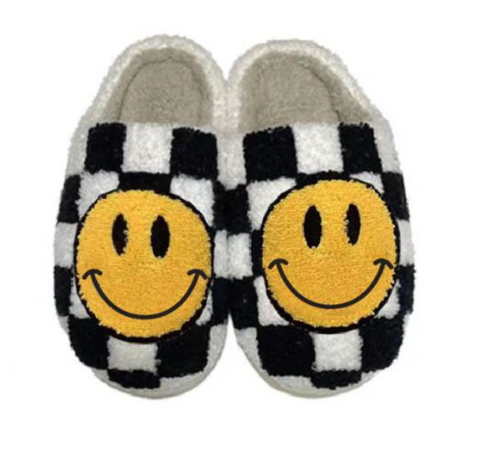# Assorted Slippers

• \$26.00

Our Size Breakdown:

S: euro 37/38

M: euro 39/40

L: euro 41/42

XL: euro 43/44

Actual Size Breakdown:

36/37 = 6

37 = 6.5

37/38 =7

38 = 7.5

38/39 = 8

39 = 8.5

39/40=9

40 = 9.5

40/41 = 10

41 = 10.5

41/42 = 11

42 = 11.5